# Permutations and Combinations CSAPMA 202 Epp section 6

• Slides: 21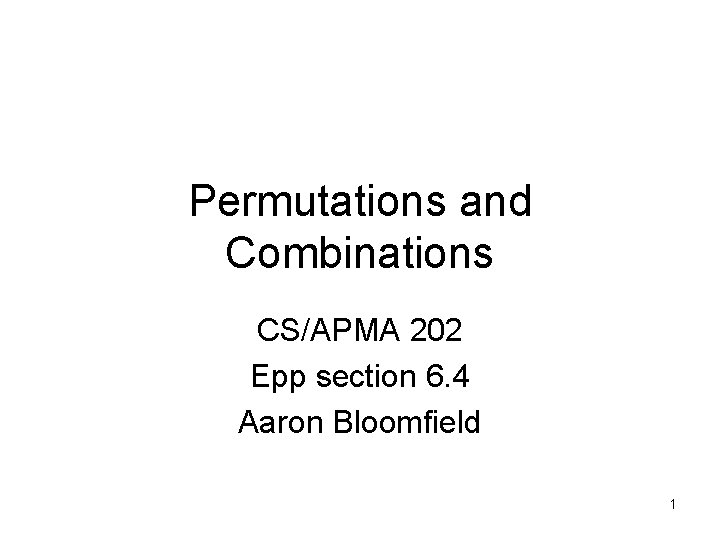Permutations and Combinations CS/APMA 202 Epp section 6. 4 Aaron Bloomfield 1Permutations vs. Combinations • Both are ways to count the possibilities • The difference between them is whether order matters or not • Consider a poker hand: – A♦, 5♥, 7♣, 10♠, K♠ • Is that the same hand as: – K♠, 10♠, 7♣, 5♥, A♦ • Does the order the cards are handed out matter? – If yes, then we are dealing with permutations – If no, then we are dealing with combinations 2Permutations • A permutation is an ordered arrangement of the elements of some set S – Let S = {a, b, c} – c, b, a is a permutation of S – b, c, a is a different permutation of S • An r-permutation is an ordered arrangement of r elements of the set – A♦, 5♥, 7♣, 10♠, K♠ is a 5 -permutation of the set of cards • The notation for the number of r-permutations: P(n, r) – The poker hand is one of P(52, 5) permutations 3Permutations • Number of poker hands (5 cards): – P(52, 5) = 52*51*50*49*48 = 311, 875, 200 • Number of (initial) blackjack hands (2 cards): – P(52, 2) = 52*51 = 2, 652 • r-permutation notation: P(n, r) – The poker hand is one of P(52, 5) permutations 4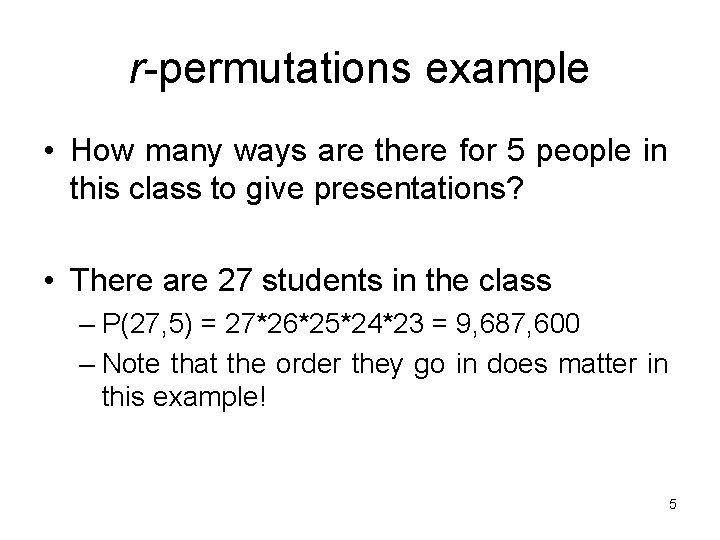r-permutations example • How many ways are there for 5 people in this class to give presentations? • There are 27 students in the class – P(27, 5) = 27*26*25*24*23 = 9, 687, 600 – Note that the order they go in does matter in this example! 5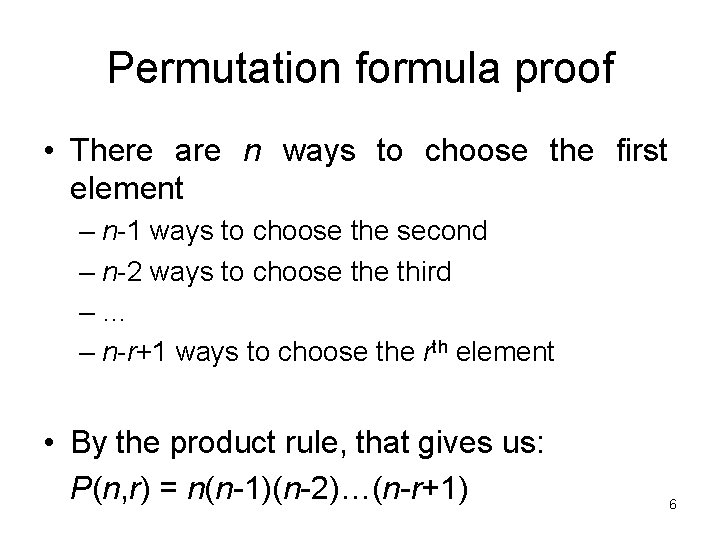Permutation formula proof • There are n ways to choose the first element – n-1 ways to choose the second – n-2 ways to choose third –… – n-r+1 ways to choose the rth element • By the product rule, that gives us: P(n, r) = n(n-1)(n-2)…(n-r+1) 6Permutations vs. r-permutations • r-permutations: Choosing an ordered 5 card hand is P(52, 5) – When people say “permutations”, they almost always mean r-permutations • But the name can refer to both • Permutations: Choosing an order for all 52 cards is P(52, 52) = 52! – Thus, P(n, n) = n! 7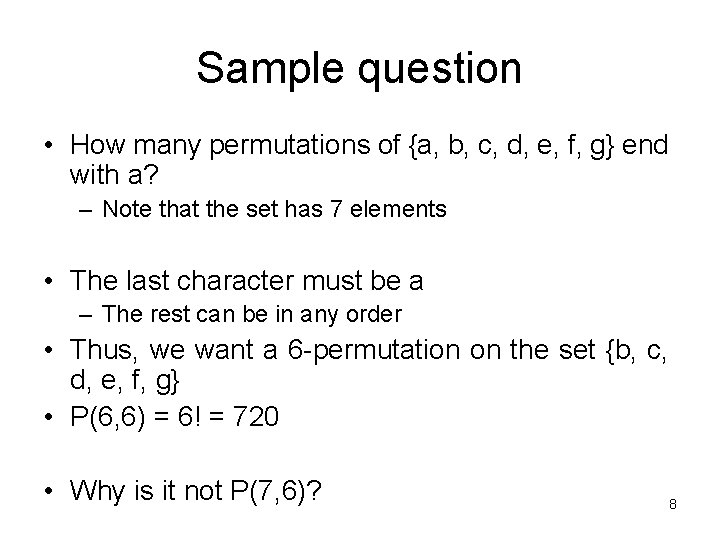Sample question • How many permutations of {a, b, c, d, e, f, g} end with a? – Note that the set has 7 elements • The last character must be a – The rest can be in any order • Thus, we want a 6 -permutation on the set {b, c, d, e, f, g} • P(6, 6) = 6! = 720 • Why is it not P(7, 6)? 8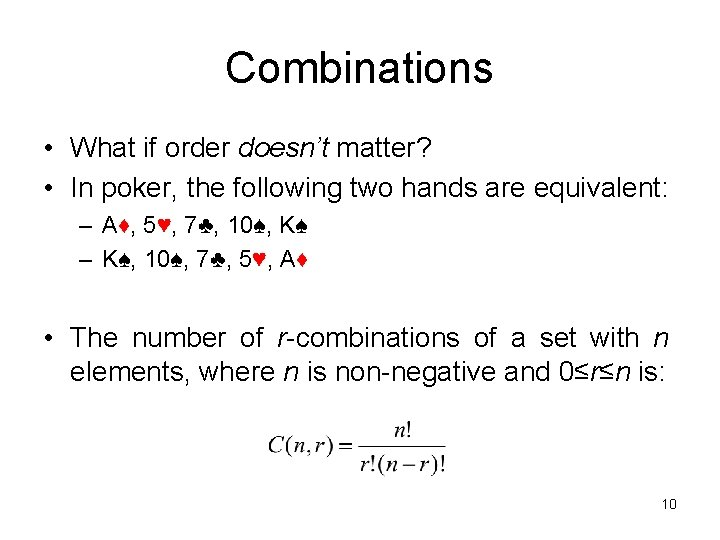Combinations • What if order doesn’t matter? • In poker, the following two hands are equivalent: – A♦, 5♥, 7♣, 10♠, K♠ – K♠, 10♠, 7♣, 5♥, A♦ • The number of r-combinations of a set with n elements, where n is non-negative and 0≤r≤n is: 10Combinations example • How many different poker hands are there (5 cards)? • How many different (initial) blackjack hands are there? 11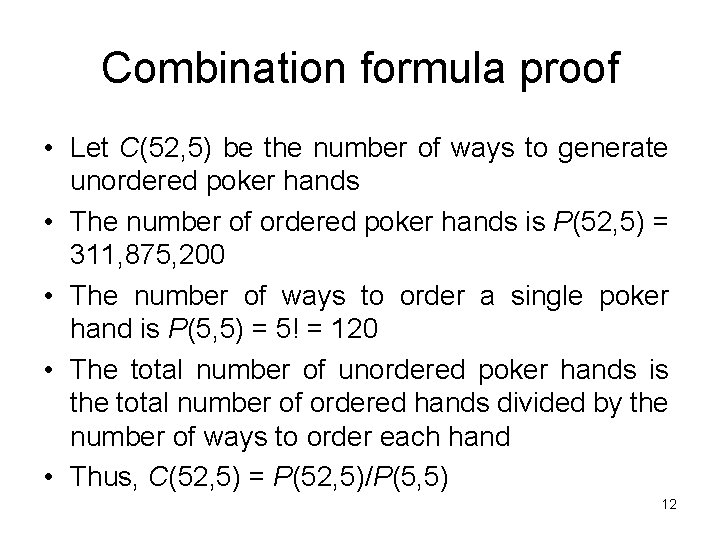Combination formula proof • Let C(52, 5) be the number of ways to generate unordered poker hands • The number of ordered poker hands is P(52, 5) = 311, 875, 200 • The number of ways to order a single poker hand is P(5, 5) = 5! = 120 • The total number of unordered poker hands is the total number of ordered hands divided by the number of ways to order each hand • Thus, C(52, 5) = P(52, 5)/P(5, 5) 12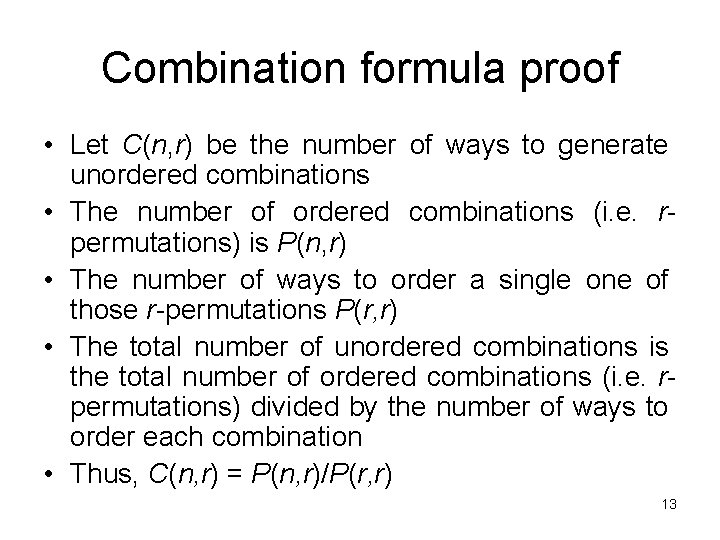Combination formula proof • Let C(n, r) be the number of ways to generate unordered combinations • The number of ordered combinations (i. e. rpermutations) is P(n, r) • The number of ways to order a single one of those r-permutations P(r, r) • The total number of unordered combinations is the total number of ordered combinations (i. e. rpermutations) divided by the number of ways to order each combination • Thus, C(n, r) = P(n, r)/P(r, r) 13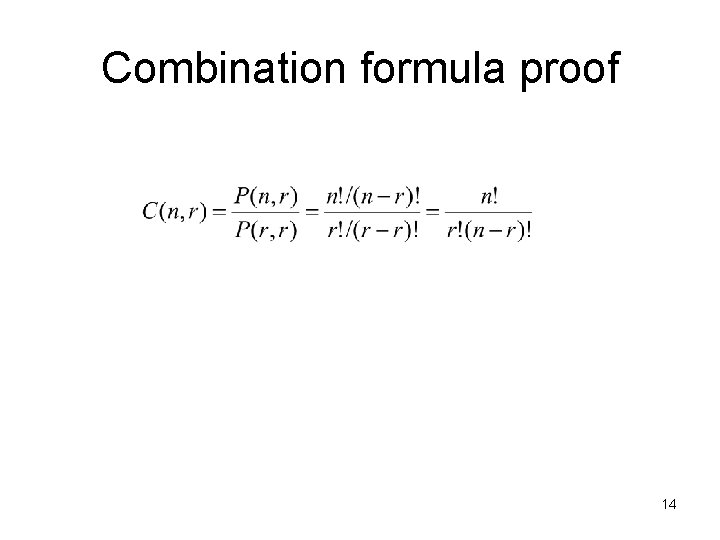Combination formula proof 14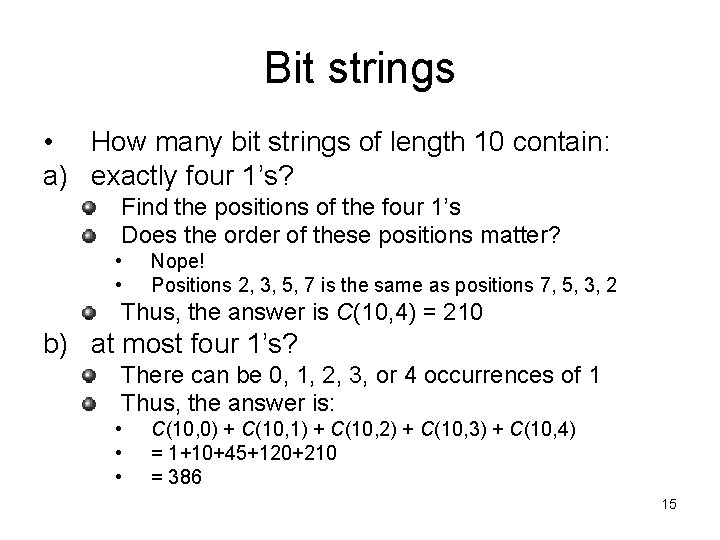Bit strings • How many bit strings of length 10 contain: a) exactly four 1’s? Find the positions of the four 1’s Does the order of these positions matter? • • Nope! Positions 2, 3, 5, 7 is the same as positions 7, 5, 3, 2 Thus, the answer is C(10, 4) = 210 b) at most four 1’s? There can be 0, 1, 2, 3, or 4 occurrences of 1 Thus, the answer is: • • • C(10, 0) + C(10, 1) + C(10, 2) + C(10, 3) + C(10, 4) = 1+10+45+120+210 = 386 15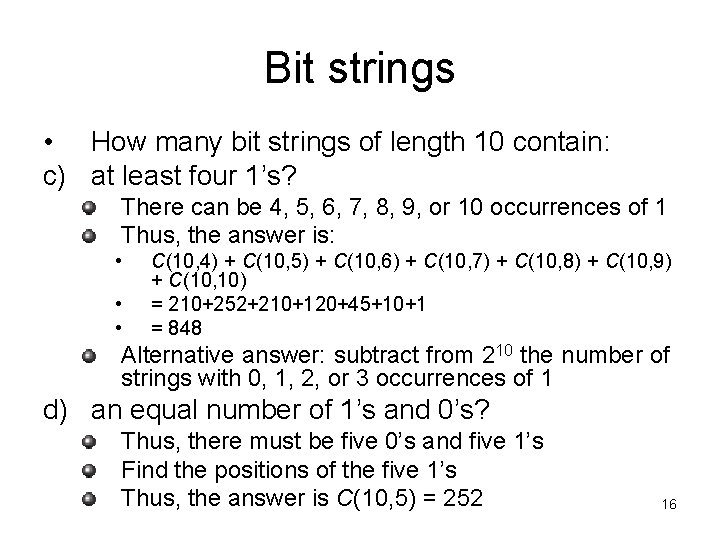Bit strings • How many bit strings of length 10 contain: c) at least four 1’s? There can be 4, 5, 6, 7, 8, 9, or 10 occurrences of 1 Thus, the answer is: • • • C(10, 4) + C(10, 5) + C(10, 6) + C(10, 7) + C(10, 8) + C(10, 9) + C(10, 10) = 210+252+210+120+45+10+1 = 848 Alternative answer: subtract from 210 the number of strings with 0, 1, 2, or 3 occurrences of 1 d) an equal number of 1’s and 0’s? Thus, there must be five 0’s and five 1’s Find the positions of the five 1’s Thus, the answer is C(10, 5) = 252 16Corollary 1 • Let n and r be non-negative integers with r ≤ n. Then C(n, r) = C(n, n-r) • Proof: 17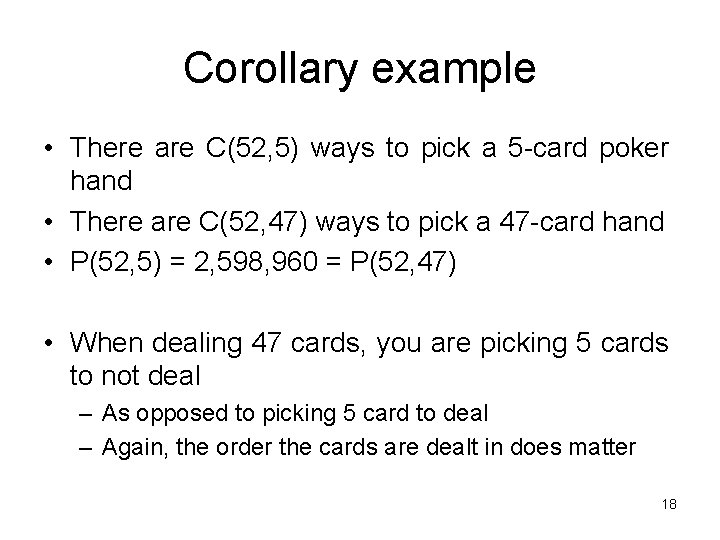Corollary example • There are C(52, 5) ways to pick a 5 -card poker hand • There are C(52, 47) ways to pick a 47 -card hand • P(52, 5) = 2, 598, 960 = P(52, 47) • When dealing 47 cards, you are picking 5 cards to not deal – As opposed to picking 5 card to deal – Again, the order the cards are dealt in does matter 18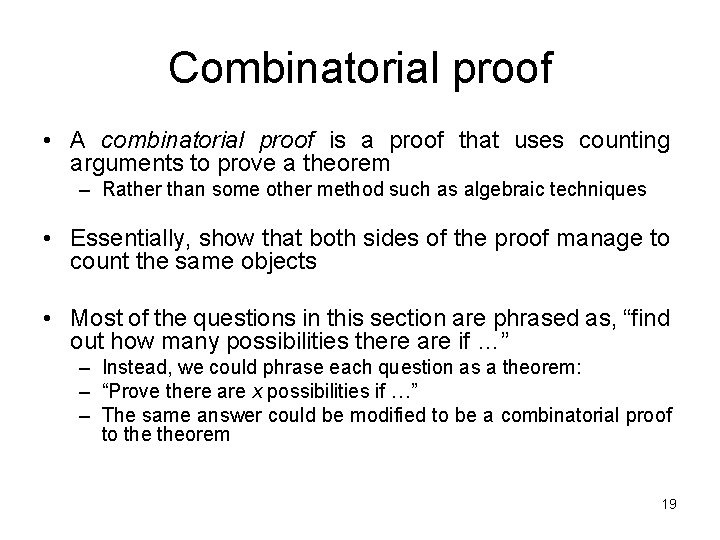Combinatorial proof • A combinatorial proof is a proof that uses counting arguments to prove a theorem – Rather than some other method such as algebraic techniques • Essentially, show that both sides of the proof manage to count the same objects • Most of the questions in this section are phrased as, “find out how many possibilities there are if …” – Instead, we could phrase each question as a theorem: – “Prove there are x possibilities if …” – The same answer could be modified to be a combinatorial proof to theorem 19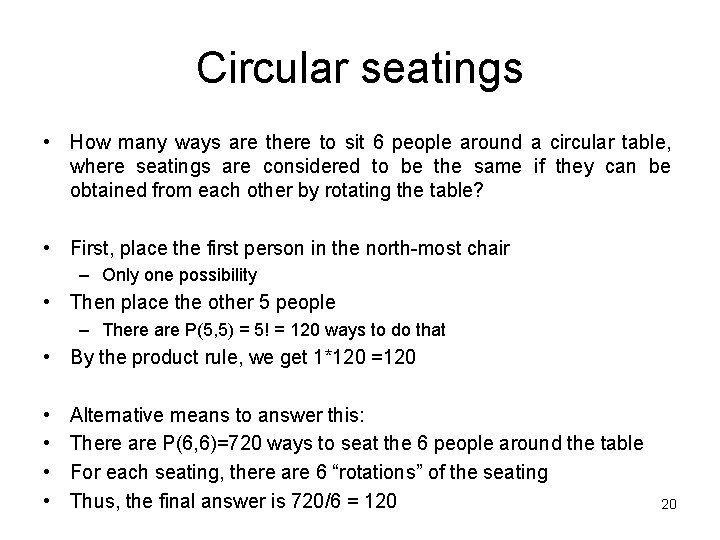Circular seatings • How many ways are there to sit 6 people around a circular table, where seatings are considered to be the same if they can be obtained from each other by rotating the table? • First, place the first person in the north-most chair – Only one possibility • Then place the other 5 people – There are P(5, 5) = 5! = 120 ways to do that • By the product rule, we get 1*120 =120 • • Alternative means to answer this: There are P(6, 6)=720 ways to seat the 6 people around the table For each seating, there are 6 “rotations” of the seating Thus, the final answer is 720/6 = 120 20Horse races • How many ways are there for 4 horses to finish if ties are allowed? – Note that order does matter! • Solution by cases – No ties • The number of permutations is P(4, 4) = 4! = 24 – Two horses tie • There are C(4, 2) = 6 ways to choose the two horses that tie • There are P(3, 3) = 6 ways for the “groups” to finish – A “group” is either a single horse or the two tying horses • By the product rule, there are 6*6 = 36 possibilities for this case – Two groups of two horses tie • There are C(4, 2) = 6 ways to choose the two winning horses • The other two horses tie for second place – Three horses tie with each other • There are C(4, 3) = 4 ways to choose the two horses that tie • There are P(2, 2) = 2 ways for the “groups” to finish • By the product rule, there are 4*2 = 8 possibilities for this case – All four horses tie • There is only one combination for this – By the sum rule, the total is 24+36+6+8+1 = 75 21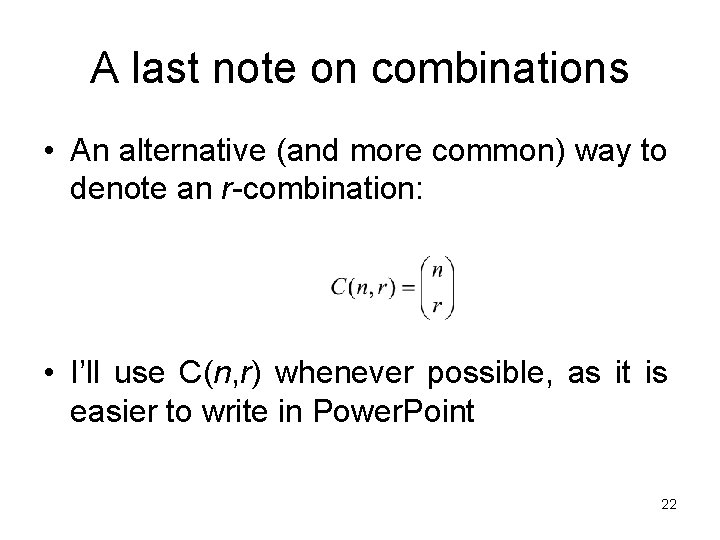A last note on combinations • An alternative (and more common) way to denote an r-combination: • I’ll use C(n, r) whenever possible, as it is easier to write in Power. Point 22